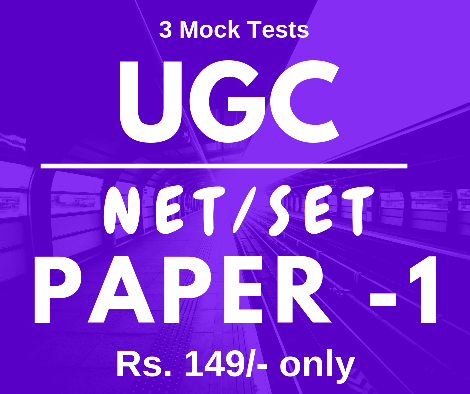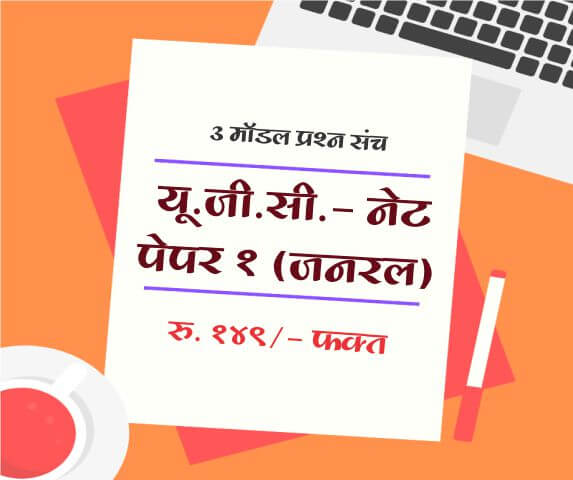# PERCENTAGE - (ENGLISH)

Share Now

Practicing Percentage for Aptitude Test: Tricks and Shortcuts

Quantitative Aptitude is an important section in almost all competitive exams. It helps to determine the candidate’s ability to deal with the numbers and process them with higher accuracy. So many topics are covered in this section; however, here we are going to talk about Percentage; one of the high scoring parts in any quantitative aptitude exam.

A wide range of questions in percentage category is related to the successive change in the percentage of change of percentage. Let us consider a general example “In an electronics shop, price of a refrigerator is marked at \$1000, it was discounted at 20% on Christmas eve and then further discounted by 30% on New Year eve. Calculate the price of the refrigerator at present?” Here, candidates need to calculate the successive change in percentage to determine the final discounted price of the commodity.

Although, most of the questions in the percentage section use to be quite simple and easier to solve; those who are afraid of mathematics may find them little difficult to solve. Don’t worry! Below we have highlighted a few tips, concepts, and tricks to solve percentage related problem in aptitude test questions.

Solving percentage related problems in career aptitude test:

In general terms, percentage means calculating something “out of one hundred” or in simple words “per hundred.” It is the basic technique of representing a part-to-whole relationship that is a useful mathematical expression to calculate how many portions or parts one has obtained out of the whole thing. For example, two glasses of water out of whole 8 glasses can be represented as 2/8; while making calculation out of 100, it can be written as 25/100 or 25%.

Percentage allows us to express the part to the whole relationship in the form of a whole number without using the decimal number system.  For instance, when we say “30% of students”, it means 30 out of every 100 students. If we talk about decimal form, it can be written as 0.25, and this fraction is also equivalent to 25/100. In simple terms, all these forms of expression represent the same piece of information.

Things to know:

• Percentage and Conversion type problems:

In order to solve questions that are in the format of “What is x% of y?” Now, in order to solve this problem, first of all, each symbol or world of this sentence must be translated into mathematical terms. Here, “what” represents a variable; for example, x. The verb “is, was or are” always represent the equal sign. For the percentage symbol, the fraction must be written with respect to 100. Finally, the “of” word represent multiplication.

• Percent change type of problems:

Percent decrease or increase is the best method to represent a change in some given number. Note that, in this case, we are focusing on a single change; in case of multiple changes, we need to work on a continuous percentage change concept. Percentage increase represents the percentage in which the original number increases whereas in case of a decrease in percentage, the original number decreases.

Candidates preparing for following competitive exams can utilize Aptitude section to improve their skills.

• Bank Competitive Exam
• MPSC Competitive Exams
• SSC Competitive Exams
• L.I.C/ G. I.C Competitive Exams
• Railway Competitive Exam
• University Grants Commission (UGC)
• NET/ SET Competitive Exam
• Common Aptitude Test (CAT)
• Career Aptitude Test (IT Companies)

#### PERCENTAGE - (ENGLISH) is useful for following exam preparation

 Quantitative Aptitude for Competitive Exam IBPS Bank Exam Maharashtra B.Ed. CET AP LAWCET TSLAWCET AILET IBPS RRB MPSC Sales tax Inspector UPTET RBI Assistant SBI Clerk MPSC State Service MPSC: Assistant Motor Vehicle Inspector Examination MPSC State Excise Sub-Inspector CLAT SSC-CGL MBA Entrance MPSC Competitive Exams Bank Aptitude Test SSC GD (Constable) Recruitment

#### Mock Exam Papers

Latest Articles
Popular Articles
Upcoming Exam Forms

Full Length Mock Tests
Timer Based Exams
Instant Result and assesment
Detailed analasys of Result##### UGC NET SET Paper 1 Mock Tests

UGC-NET / SET Paper 1 mock test

3 Mock Tests, 150 Questions with Explanations

Rs: 149 Only/-##### LAW MH-CET 2019 (3 YEAR COURSE)

Mock test for MH CET Law 2019 for three year law programme

3 Mock Tests, 450 Questions with Explanations

Rs: 249 Only/-##### यू. जी. सी. - नेट पेपर १ (जनरल)

यू. जी. सी. - नेट पेपर १ (अनिवार्य) सामान्य प्रश्न हिंदीमें

3 Mock Tests, 150 Questions with Explanations

Rs: 149 Only/-